# Electronic configurationPage 1

#### WATCH ALL SLIDES

Slide 1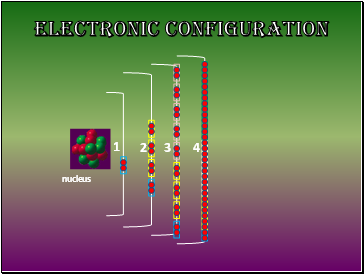Electronic Configuration

nucleus

Slide 2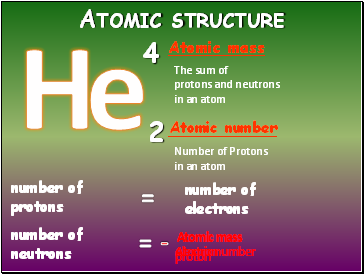## Atomic structure

4

Atomic mass

2

Atomic number

number of

electrons

number of

protons

=

The sum of

protons and neutrons

in an atom

Number of Protons

in an atom

Atomic mass

Atomic number

-

Atomic mass

proton

-

Atomic mass

electron

-

Slide 3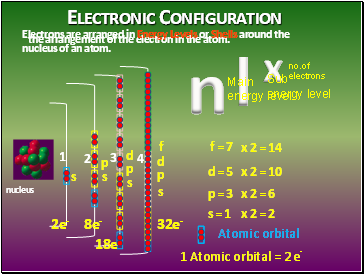## Electronic Configuration

the arrangement of the electron in the atom.

Electrons are arranged in Energy Levels or Shells around the nucleus of an atom.

nucleus

n

l

x

s

d

f

s

p

s

p

d

p

s

Atomic orbital

f = 7

d = 5

p = 3

s = 1

1 Atomic orbital = 2 e-

x 2 = 2

x 2 = 6

x 2 = 10

x 2 = 14

2e-

8e-

32e-

Main energy level

Sub

energy level

no.of

electrons

18e-

Slide 4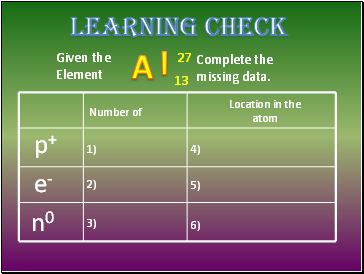## Learning Check

Given the

Element

Complete the

missing data.

p+

e-

n0

Number of

Location in the

atom

1)

2)

3)

4)

5)

6)

Slide 5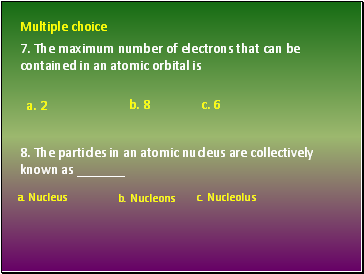7. The maximum number of electrons that can be contained in an atomic orbital is

8. The particles in an atomic nucleus are collectively known as _

Multiple choice

a. 2

a. Nucleus c. Nucleolus

b. Nucleons

b. 8 c. 6

Slide 6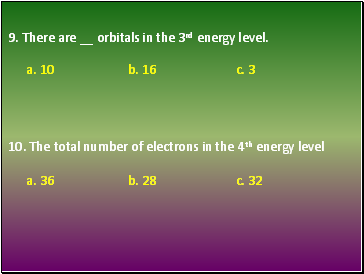9. There are orbitals in the 3rd energy level.

10. The total number of electrons in the 4th energy level

a. 10 b. 16 c. 3

a. 36 b. 28 c. 32

Slide 7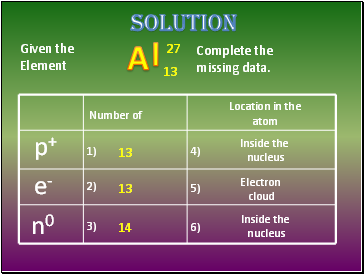Given the

Element

Complete the

missing data.

p+

e-

n0

Number of

Location in the

atom

1)

2)

3)

4)

5)

6)

solution

13

13

14

Inside the

nucleus

Inside the

nucleus

Electron

cloud

Slide 8## Multiple choice

a. Nucleus c. Nucleolus

7. The maximum number of electrons that can be contained in an atomic orbital is

8. The particles in an atomic nucleus are collectively known as _

b. 8 c. 6

b. Nucleons

a. 2

Slide 9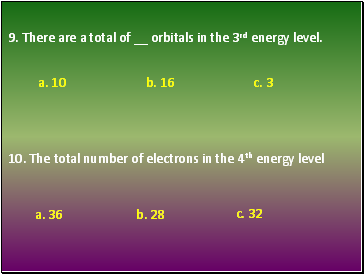9. There are a total of orbitals in the 3rd energy level.

10. The total number of electrons in the 4th energy level

Go to page:
1  2